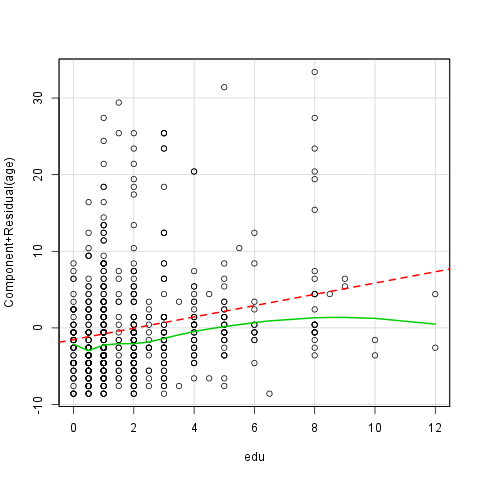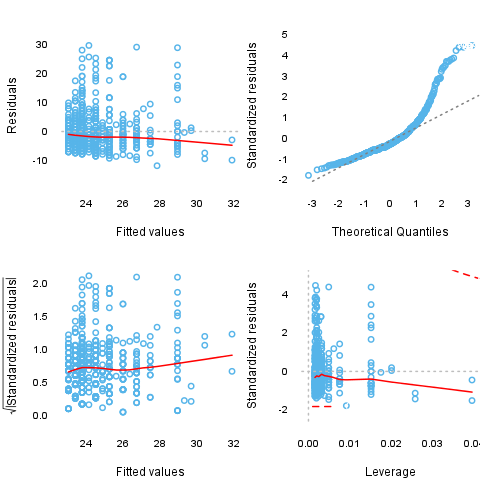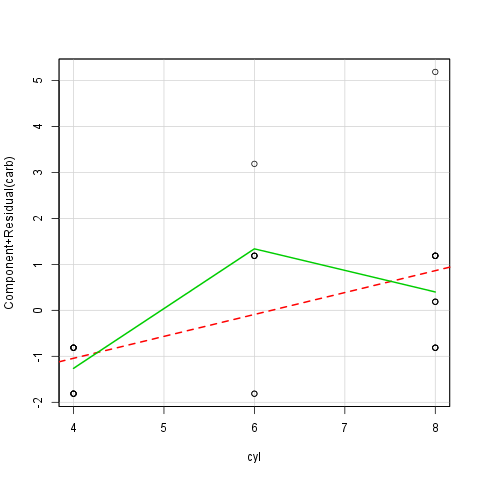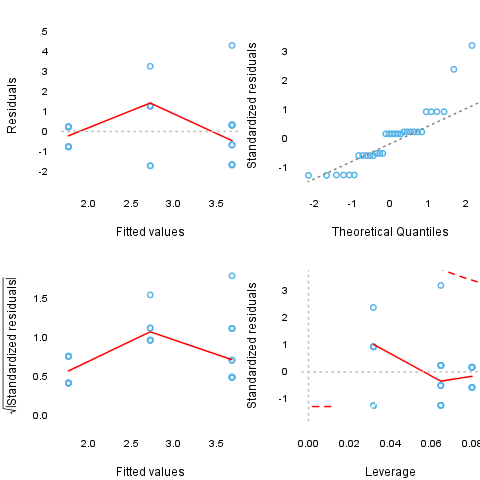## Description

This template will run a Linear Regression

### Introduction

With the help of the linear regression we can investigate the relationship between two variables. More punctually we can observe if one of the variables, the so-called dependent variable, significantly depended on the other variable, if an increase/decrease on the dependent variable's values made an increase/decrease on the independent variable. In this case we only observe linear relationships.

### Overview

Linear Regression was carried out, with Internet usage for educational purposes (hours per day) as independent variable, and Age as a dependent variable.

### Assumptions

In order to have reliable results, we have to check if the assumptions of the linear regression met with the data we used.

Table continues below
Value p-value
Global Stat 875.9 0
Skewness 378.9 0
Kurtosis 490.7 0
Heteroscedasticity 5.404 0.0201
Decision
Global Stat Assumptions NOT satisfied!
Skewness Assumptions NOT satisfied!
Kurtosis Assumptions NOT satisfied!
Heteroscedasticity Assumptions NOT satisfied!

To check these assumptions the so-called GVLMA, the Global Validation of Linear Model Assumptions will help us. The result of that we can see in the table above.

The GVLMA makes a thorough detection on the linear model, including tests generally about the fit, the shape of the distribution of the residuals (skewness and kurtosis), the linearity and the homoskedasticity. On the table we can see if our model met with the assumptions. As a generally accepted thumb-rule we use the critical p-value=0.05.

So let's see the results, which the test gave us:

• The general statistic tells us about the linear model, that it does not fit to our data.

• According to the GVLMA the residuals of our model's skewness differs significantly from the normal distribution's skewness.

• The residuals of our model's kurtosis differs significantly from the normal distribution's kurtosis, based on the result of the GVLMA.

• In the row of the link function we can read that the linearity assumption of our model was accepted.

• At last but not least GVLMA confirms the violation of homoscedasticity.

In summary: We can 't be sure that the linear model used here fits to the data.

References:

• Pena, EA and Slate, EH (2006): Global validation of linear model assumptions. J. Amer. Statist. Assoc. 101 (473):341-354.
##### Nonlinearity

As we want to fit a linear regression model, it is advisable to see if the relationship between the used variables are linear indeed. Next to the test statistic of the GVLMA it is advisable to use a graphical device as well to check that linearity. Here we will use the so-called crPlots funtion to do that, which is an abbreviation of the Component + Residual Plot.Here comes the question: What do we see on the plot? First of all we can see two lines and several circles. The red interrupted line is the best fitted linear line, which means that te square of the residuals are the least while fitting that line in the model. The green curved line is the best fitted line, which does not have to be straight, of all. The observations we investigate are the circles. We can talk about linearity if the green line did not lie too far from the red.

Next to these options there is a possibility to have a glance on the so-called diagnostic plots, which on we can see the residuals in themselves and in standardized forms.### Results

After successfully checked the assumptions we can finally turn to the main part of the interest, the results of the Linear Regression Model. From the table we can read the variables which have significant effect on the dependent variable.

Fitting linear model: age based on edu
Estimate Std. Error t value Pr(>|t|)
edu 0.7373 0.1307 5.643 2.521e-08
(Intercept) 23.07 0.3771 61.17 2.273e-268
Number of Cases Adjusted R Squared AIC BIC
637 0.04625 4231 4244

From the table one can see that (Intercept) has significant effect on the dependent variable, the p-value of that is 0 and edu has significant effect on the dependent variable, the p-value of that is 0

The model does not fit well, because the Adjusted R Square is 0.04625.

## Description

This template will run a Linear Regression

### Introduction

With the help of the linear regression we can investigate the relationship between two variables. More punctually we can observe if one of the variables, the so-called dependent variable, significantly depended on the other variable, if an increase/decrease on the dependent variable's values made an increase/decrease on the independent variable. In this case we only observe linear relationships.

### Overview

Linear Regression was carried out, with Internet usage for educational purposes (hours per day) as independent variable, and Age as a dependent variable.

### Assumptions

In order to have reliable results, we have to check if the assumptions of the linear regression met with the data we used.

Table continues below
Value p-value
Global Stat 875.9 0
Skewness 378.9 0
Kurtosis 490.7 0
Heteroscedasticity 5.404 0.0201
Decision
Global Stat Assumptions NOT satisfied!
Skewness Assumptions NOT satisfied!
Kurtosis Assumptions NOT satisfied!
Heteroscedasticity Assumptions NOT satisfied!

To check these assumptions the so-called GVLMA, the Global Validation of Linear Model Assumptions will help us. The result of that we can see in the table above.

The GVLMA makes a thorough detection on the linear model, including tests generally about the fit, the shape of the distribution of the residuals (skewness and kurtosis), the linearity and the homoskedasticity. On the table we can see if our model met with the assumptions. As a generally accepted thumb-rule we use the critical p-value=0.05.

So let's see the results, which the test gave us:

• The general statistic tells us about the linear model, that it does not fit to our data.

• According to the GVLMA the residuals of our model's skewness differs significantly from the normal distribution's skewness.

• The residuals of our model's kurtosis differs significantly from the normal distribution's kurtosis, based on the result of the GVLMA.

• In the row of the link function we can read that the linearity assumption of our model was accepted.

• At last but not least GVLMA confirms the violation of homoscedasticity.

In summary: We can 't be sure that the linear model used here fits to the data.

References:

• Pena, EA and Slate, EH (2006): Global validation of linear model assumptions. J. Amer. Statist. Assoc. 101 (473):341-354.
##### Nonlinearity

As we want to fit a linear regression model, it is advisable to see if the relationship between the used variables are linear indeed. Next to the test statistic of the GVLMA it is advisable to use a graphical device as well to check that linearity. Here we will use the so-called crPlots funtion to do that, which is an abbreviation of the Component + Residual Plot.Here comes the question: What do we see on the plot? First of all we can see two lines and several circles. The red interrupted line is the best fitted linear line, which means that te square of the residuals are the least while fitting that line in the model. The green curved line is the best fitted line, which does not have to be straight, of all. The observations we investigate are the circles. We can talk about linearity if the green line did not lie too far from the red.

Next to these options there is a possibility to have a glance on the so-called diagnostic plots, which on we can see the residuals in themselves and in standardized forms.### Results

After successfully checked the assumptions we can finally turn to the main part of the interest, the results of the Linear Regression Model. From the table we can read the variables and interactions which have significant effect on the dependent variable.

Fitting linear model: age based on edu
Estimate Std. Error t value Pr(>|t|)
edu 0.7373 0.1307 5.643 2.521e-08
(Intercept) 23.07 0.3771 61.17 2.273e-268
Number of Cases Adjusted R Squared AIC BIC
637 0.04625 4231 4244

From the table one can see that (Intercept) has significant effect on the dependent variable, the p-value of that is 0 and edu has significant effect on the dependent variable, the p-value of that is 0

The model does not fit well, because the Adjusted R Square is 0.04625.

## Description

This template will run a Linear Regression

### Introduction

With the help of the linear regression we can investigate the relationship between two variables. More punctually we can observe if one of the variables, the so-called dependent variable, significantly depended on the other variable, if an increase/decrease on the dependent variable's values made an increase/decrease on the independent variable. In this case we only observe linear relationships.

### Overview

Linear Regression was carried out, with cyl as independent variable, and carb as a dependent variable.

### Assumptions

In order to have reliable results, we have to check if the assumptions of the linear regression met with the data we used.

Table continues below
Value p-value
Global Stat 19.91 0.0005211
Skewness 7.299 0.006899
Kurtosis 5.033 0.02486
Heteroscedasticity 5.124 0.0236
Decision
Global Stat Assumptions NOT satisfied!
Skewness Assumptions NOT satisfied!
Kurtosis Assumptions NOT satisfied!
Heteroscedasticity Assumptions NOT satisfied!

To check these assumptions the so-called GVLMA, the Global Validation of Linear Model Assumptions will help us. The result of that we can see in the table above.

The GVLMA makes a thorough detection on the linear model, including tests generally about the fit, the shape of the distribution of the residuals (skewness and kurtosis), the linearity and the homoskedasticity. On the table we can see if our model met with the assumptions. As a generally accepted thumb-rule we use the critical p-value=0.05.

So let's see the results, which the test gave us:

• The general statistic tells us about the linear model, that it does not fit to our data.

• According to the GVLMA the residuals of our model's skewness differs significantly from the normal distribution's skewness.

• The residuals of our model's kurtosis differs significantly from the normal distribution's kurtosis, based on the result of the GVLMA.

• In the row of the link function we can read that the linearity assumption of our model was accepted.

• At last but not least GVLMA confirms the violation of homoscedasticity.

In summary: We can 't be sure that the linear model used here fits to the data.

References:

• Pena, EA and Slate, EH (2006): Global validation of linear model assumptions. J. Amer. Statist. Assoc. 101 (473):341-354.
##### Nonlinearity

As we want to fit a linear regression model, it is advisable to see if the relationship between the used variables are linear indeed. Next to the test statistic of the GVLMA it is advisable to use a graphical device as well to check that linearity. Here we will use the so-called crPlots funtion to do that, which is an abbreviation of the Component + Residual Plot.Here comes the question: What do we see on the plot? First of all we can see two lines and several circles. The red interrupted line is the best fitted linear line, which means that te square of the residuals are the least while fitting that line in the model. The green curved line is the best fitted line, which does not have to be straight, of all. The observations we investigate are the circles. We can talk about linearity if the green line did not lie too far from the red.

Next to these options there is a possibility to have a glance on the so-called diagnostic plots, which on we can see the residuals in themselves and in standardized forms.### Results

After successfully checked the assumptions we can finally turn to the main part of the interest, the results of the Linear Regression Model. From the table we can read the variables which have significant effect on the dependent variable.

Fitting linear model: carb based on cyl
Estimate Std. Error t value Pr(>|t|)
cyl 0.4766 0.1403 3.396 0.001942
(Intercept) -0.1365 0.9027 -0.1513 0.8808
Number of Cases Adjusted R Squared AIC BIC
32 0.2536 116.1 120.5

From the table one can see that cyl has significant effect on the dependent variable, the p-value of that is 0.002

The model fits well, because the Adjusted R Square is 0.2536.

This report was generated with R (3.0.1) and rapport (0.51) in 3.731 sec on x86_64-unknown-linux-gnu platform.